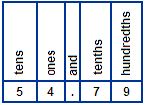Adding and Subtracting Decimals

Adding and Subtracting Decimals is a unit of instruction that shows students how to read, write, compare, order, add and subtract decimals. Other topics include estimating decimal sums and differences, and solving word problems. Try our sample lessons below, or browse other instructional units.Topics Description Introduction to Decimals Students are introduced to decimal numbers as they relate to place value. Expanded form and decimal digits are also covered. Reading and Writing Decimals Students are shown how to read and write decimals. They learn to write mixed numbers and phrases as decimals, and to read and write decimals using words. Comparing Decimals Students are shown how to compare decimals using the correct symbols. Ordering Decimals Students are shown how to order three or more decimals from least to greatest. Estimating Decimal Sums This lesson shows students how to estimate decimal sums using several estimation strategies. Overestimates and underestimates are also covered. Adding Decimals Step-by-step examples for adding two or decimals are presented. Estimating Decimal Differences This lesson shows students how to estimate decimal differences by rounding to various places. Overestimates and underestimates are also covered. Subtracting Decimals Step-by-step examples for subtracting decimals are presented. Solving Decimal Word Problems Students are shown how to solve decimal word problems using the concepts learned in this unit. Practice Exercises To complete 10 additional exercises as practice. To assess students' understanding of all concepts learned so far. Challenge Exercises To solve 10 additional problems that challenge students' understanding of all concepts presented in this unit. To hone students' problem-solving skills. Solutions To review complete solutions to all exercises presented in this unit. Includes the problem, step-by-step solutions, final answer for each exercise.